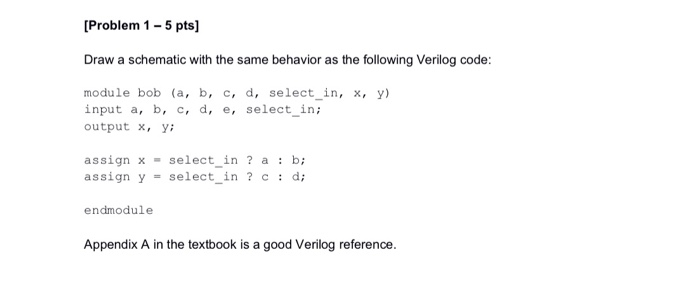# Question [Problem 1 - 5 pts) Draw a schematic with the same behavior as the following Verilog code: module bob (a, b, c, d, select_in, x, y) input a, b, c, d, e, select_in; output x, y; assign x = select_in ? a : b; assign y = select_in?c : d; endmodule Appendix A in the textbook is a good Verilog reference.4QPJWD The Asker · Electrical EngineeringTranscribed Image Text: [Problem 1 - 5 pts) Draw a schematic with the same behavior as the following Verilog code: module bob (a, b, c, d, select_in, x, y) input a, b, c, d, e, select_in; output x, y; assign x = select_in ? a : b; assign y = select_in?c : d; endmodule Appendix A in the textbook is a good Verilog reference.
More
Transcribed Image Text: [Problem 1 - 5 pts) Draw a schematic with the same behavior as the following Verilog code: module bob (a, b, c, d, select_in, x, y) input a, b, c, d, e, select_in; output x, y; assign x = select_in ? a : b; assign y = select_in?c : d; endmodule Appendix A in the textbook is a good Verilog reference.